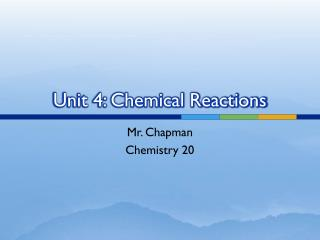DownloadDownload PresentationUnit 4: Chemical Reactions

# Unit 4: Chemical Reactions

Download Presentation## Unit 4: Chemical Reactions

- - - - - - - - - - - - - - - - - - - - - - - - - - - E N D - - - - - - - - - - - - - - - - - - - - - - - - - - -
##### Presentation Transcript

1. Unit 4: Chemical Reactions Mr. Chapman Chemistry 20

2. Old School Chemistry Reviewing Grade 10

3. What is a Chemical Reaction? • In a chemical reaction, substances react with each other to form completely new substances. • There are several common indicators that a person can look at to know that a chemical reaction has occurred. Chemical Reactions

4. Indicators of a Chemical Reaction • Heat or light is given off, or heat is absorbed. • Colour change • A solid is formed • A gas is formed • The change is difficult to reverse

5. Examples: • Observe the two examples of a chemical reaction. • The first chemical reaction is between barium hydroxide and ammonium thiocyanate. • The second reaction is between sucrose and sulfuric acid. • Record your observations after watching the reactions. How many indicators of reactions did you see?

6. Word Equations • A word equation is one way of representing a chemical reaction. A word equation tells you what reacts and what is produced. • Word equations allow chemists to recognize patterns, and to predict what the products of a chemical reaction might be. • Word equations are written in a specific format.

7. Word Equation Format • The left side of the equation represents all of the reactants. The reactants are the chemicals that combine to form a new substance. • The right side of the equation contains all of the products. The products are the result of the chemical reaction. • An arrow points from the reactants to the products.

8. Word Equations from Examples First Reaction • Barium hydroxide + Ammonium thiocyanate • Ammonia gas + Water + Barium thiocyanate Second Reaction • Sucrose + Sulfuric acid  Carbon + Water

9. Word Equations • Notice that ALL reactants and products are separated by plus signs. We never write a minus sign in an equation, it does not make any sense in chemistry. All the reactants  All the productsreactant 1 + reactant 2  product 1 + product 2

10. Examples: • Iron reacts with oxygen in the air to form rust, also known as iron (III) oxide. Write a word equation that represents this change. • Copper is placed in a beaker of silver nitrate, forming silver metal and copper(II) nitrate. Write a word equation that represents this change. • Hydrogen is produced when zinc metal and hydrochloric acid react with each other, and zinc chloride is also formed.Write a word equation that represents this change.

11. Skeleton Equations • Skeleton equationsare also used to represent chemical reactions, but instead of using words to present the chemicals, the formulas are used. • Word Equation: hydrogen + oxygen  water • Skeleton Equation: H2 + O2H2O

12. Skeleton Equations • If you understand how to write a word equation, you can most definitely write a skeleton equation. • Just a couple of notes: • The formula of a metal is just the symbol for that metal. For example, if sodium reacts with water, the formula for sodium is Na. • Remember about the diatomic elements. For example, when oxygen is written as a reactant or product, its formula is O2.

13. Write Some Word & Skeleton Equations • Sodium reacts with chlorine to form sodium chloride • Copper and water are formed when copper (II) oxide reacts with hydrogen • Hydrogen and chlorine react to form hydrochloric acid (HCl) • Lead and sulfur dioxide are formed when lead (II) sulfide and oxygen react. • The product of a reaction between aluminum metal and bromine is aluminum bromide. • Hydrogen sulfide decomposes to form hydrogen and sulfur (the formula for sulfur is S8). • Lead (II) nitrate and potassium iodide combine to form lead (II) iodide and potassium nitrate. • Carbon monoxide and oxygen react to produce carbon dioxide. • Hydrogen gas and calcium hydroxide are produced when calcium reacts with water.